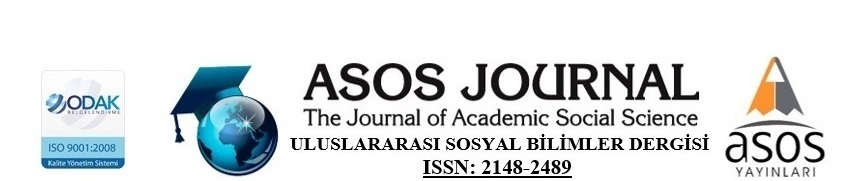### STATIC AND DYNAMIC PREDICTIONS OF RISK VALUES (VaR) OF BANK STOCKS : PEAKS – OVER – THRESHOLD MODEL AND GENERALIZED AUTOREGRESSIVE SCORE MODELS

The calculation of the value at risk of assets is a frequently used means of measuring financial risk. Risk Values may be valued and interpreted within the framework of extreme value theory because of excessive values due to uncertainties in returns. In this study, the static and dynamic VaR forecasts of the stocks of five banks operating in developed and developing country markets are determined using the POT and GAS models, respectively. The number of extreme values required for the estimation of the POT model was calculated separately using square root and 10% of the total number of data, which are two practical methods in the literature. The results were interpreted separately. In addition, GAS models based on normal, student-t and asymmetric student-t distributions were estimated and compared to each other.

#### Keywords

value at risk, POT, GAS, extreme values, forecasting

Author : Engin BEKAR
Number of pages: 142-169
DOI: http://dx.doi.org/10.29228/ASOS.36752
Full text:The Journal of Academic Social Sciences